# SSC CGL EXAMS 2018 | Reasoning Ability Practice Questions (Day-7)

Dear Aspirants, Here we have given the Important SSC Exam 2018 Practice Test Papers. Candidates those who are preparing for SSC 2018 can practice these questions to get more confidence to Crack SSC 2018 Examination.

[WpProQuiz 4137]

Directions (1-3): A series is given with one term missing. Select the correct alternative from the given ones that will complete the series.

a) Terror

b) Display

c) Dome

d) Scolding

2) AbC, dEfG, hIjKl, Mn0pQr, ?

a) StUvWxY

b) StUvWx

c) StUvWxYZ

d) sTuVwXy

3) 1, 0.125, 1/27, 1/64, ?, 1/216

a) 0.025

b) 1/8

c) 1/128

d) 0.008

4) Harish’s birthday is on Monday 5th June. On what day of the week will be Tushar’s birthday in the same year if Tushar was born on 11th December?

a) Sunday

b) Wednesday

c) Monday

d) Tuesday

5) The weights of 4 boxes are 40, 30, 50 and 20 kg. Which of the following cannot be the total weight (in kg) of any combination of these boxes and in a combination a box can be used only once?

a) 140

b) 130

c) 90

d) 100

6) If 19 # 13 = 3; 25 # 3 = 11; 36 # 10 = 13; then what is the value of 7 # 3?

a) 21

b) 2

c) 26

d) 39

7) A and B start running from the same point. A runs 3 km west, then turns south and runs 5 km, then turns to her right and runs 7 km. B runs 1 km south, then turns to her right and runs 10 km. Where is B with respect to A row?

a) 4 km South

b) 4 km North

c) 6 km North

d) 6 km South

8) In the question a statement is given, followed by two Arguments I and II. You have to consider the statement to be true even if it seems to be at variance from commonly known facts. You have to decide which of the given arguments, if any, is a strong argument?

Statement: Should speed breakers be banned?

Arguments: I. Yes, data shows that number of accidents increase after putting the speed breakers.

No, it teaches fast drivers a lesson.

a) If only argument I is strong

b) If only argument II is strong

c) If both arguments I and II are strong

d) If neither argument I nor II is strong

9) If a mirror is placed on the line MN, then which of the answer figures is the right image of the given figure?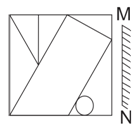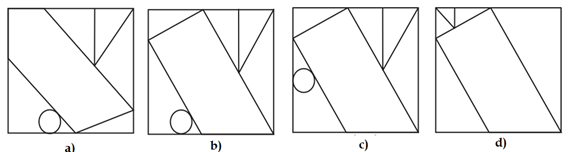10) Arrange the given words in the sequence in which they occur in the dictionary.

Exacts

Exotic

Exactly

Exacerbate

Exhaust

a) 43152

b) 43251

c) 53421

d) 54312

11) Ratio of present ages of P and Q is 9 : 4. The difference between their ages is 20 yr. What will be the sum (in yr) of their ages after 10 years?

a) 62 yr

b) 66 yr

c) 72 yr

d) 76 yr

12) In the following question, by using which mathematical operators will the expression become correct?

15 _ 3 _ 4 _ 20

a) ×, ÷ and >

b) ÷, × and <

c) ÷, × and =

d) +, × and =

13) If 19 \$ 7 = 312 and 23 \$ 9 = 448, then 31 \$ 11 = ?

a) 231

b) 441

c) 641

d) 840

14) How many triangles are there in the given figure?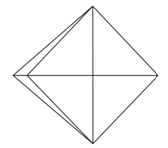15) Three positions of a cube are shown below. What will come opposite to face containing ‘N’?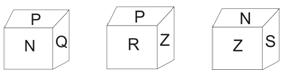Every next word is increasing by one letter, Hence, new word will be of 7 letters i/e/ new word will be of 7 letters/ i.e., display.

According to the question,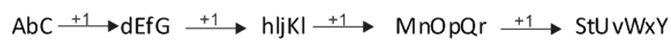Hence letter StUvWxY will come in place of question mark.

According to the question,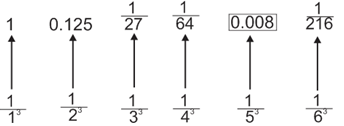Hence, 0.008 will come in place of question mark (?),

Number of days from 6th June to 11th December = 25 + 31 + 31 + 30 + 31 + 30 + 11 = 189

Number of odd days = 189  7 = 0

Weight of four boxes = 40 kg, 30 kg, 50 kg and 20 kg

Total weight of four boxes = 40 + 30 + 50 + 20 = 140

Possible combinations,

140 – 40 = 100 kg

140 – 30 = 110 kg

140 – 50 = 90 kg

140 – 20 = 120 kg

Hence, according to the options combination of 130 kg cannot be formed.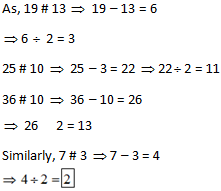Let O be the starting point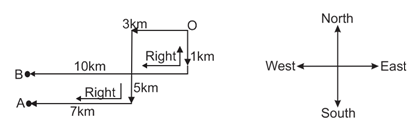Required distance = 5 – 1 = 4 km

Hence, B is 4 km faraway towards North from A.

According to the data, accidents increase after putting the speed breakers hence speed breakers should be banned because an act which is harmful must be banned. So, only argument I is strong.

On placing mirror on MN line question figure will appear like answer figure (2)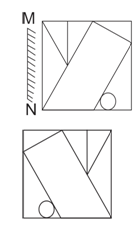According to question,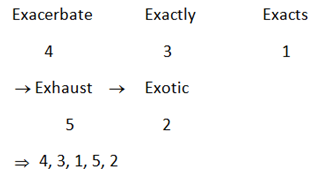Let P’s present age = 9x yr

Q’s present age = 4x yr

According to question,

9x – 4x = 20

5x = 20

x= 4

The sum of their ages after 10 yr

= 9x + 10 + 4x + 10

= 13x + 20 = 13 4 + 20

= 52 + 20 = 72 yr

Given equation, 15 – 3 – 4 – 20

From option c),

15 ÷ 3 × 4 = 20

According to BODMAS,

5 ×4 = 20

20 = 20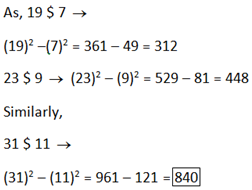According to question,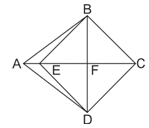Triangle, ABF, BCF, CDF, DAF, EDC, ABE, ABC, ADC, BCD, DAB

Hence, Total number of triangle = 15

R will come opposite to N.

#### *********************************